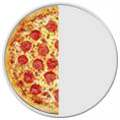# Percents (%)

### When we say "Percent" we are really saying "per 100"

One percent (1%) means 1 per 100.1% of this line is shaded green: it is very small isn't it?50% means 50 per 100 (50% of this box is green)
 25% means 25 per 100 (25% of this box is green)## Examples:100% means all. Example: 100% of 80 is 100100 × 80 = 8050% means half. Example: 50% of 80 is 50100 × 80 = 405% means 5/100ths. Example: 5% of 80 is 5100 × 80 = 4

## Using Percent

Use the slider and try some different numbers
(What is 40% of 80? What is 10% of 200? What is 90% of 10?)

### Because "Percent" means "per 100" think:

"this should be divided by 100"

So 75% really means 75100

And 100% is 100100, or exactly 1 (100% of any number is just the number, unchanged)

And 200% is 200100, or exactly 2 (200% of any number is twice the number)

### A Percent can also be expressed as a Decimal or aFractionA Half can be written... As a percentage: 50% As a decimal: 0.5 As a fraction: 1/2

## Some Worked Examples

### Example: Calculate 25% of 80

25% = 25100

And  25100 × 80 = 20

So 25% of 80 is 20

### Example: 15% of 200 apples are bad. How many apples are bad?

15% = 15100

 And  15100 × 200 =  15 × 200100 =  15 × 2 =  30 apples

### Example: if only 10 of the 200 apples are bad, what percent is that?

As a fraction, 10200 = 0.05

As a percentage it is: 10200 x 100 = 5%

5% of those apples are bad### Example: A Skateboard is reduced 25% in price in a sale. The old price was \$120. Find the new price.

First, find 25% of \$120:

25% = 25100

And  25100 × \$120 = \$30

25% of \$120 is \$30

So the reduction is \$30

Take the reduction from the original price

\$120 − \$30 = \$90

The Price of the Skateboard in the sale is \$90

## Calculation Trick

This little rule can make some calculations easier:

x% of y = y% of x

### Example: 8% of 50

8% of 50 is the same as 50% of 8

And 50% of 8 is 4

So 8% of 50 is also 4

## The Word

"Percent" comes from the latin Per Centum. The latin word Centum means 100, for example a Century is 100 years.

## Percent vs Percentage

My Dictionary says "Percentage" is the "result obtained by multiplying a quantity by a percent". So 10 percent of 50 apples is 5 apples: the 5 apples is the percentage.

But in practice people use both words the same way.